• 哈夫曼编码扩展操作码
千次阅读 多人点赞
2020-04-15 16:40:20更多相关内容
• 完全的哈夫曼编码是最优化的编码。但是这种编码的码长种类太多。如上表，7种指令就出现了4种码长，长度有1有2有3有5，不规整，不易于编码。为此，结合用一般的二进制编码，在哈夫曼编码的基础上进行扩展

2020系统结构系列文章

### 指令操作码的优化:哈夫曼编码

考完这么久了，还在搞题目，我都佩服自己。

# 指令格式

指令是由操作码地址码两部分组成的。

# 指令优化

就指令格式的优化来说,是指如何用最短的位数来表示指令的操作信息和地址信息,使程序中指令的平均字长最短。
操作码的优化，也就是最短的位数来表示指令的操作信息。

## 定长的操作码的组织方案

固定位数：比如操作码固定为6位。
在指令字最高位部分分配固定的若干位用于表示操作码，有利于简化计算机硬件设计，提高指令译码和识别速度。
例如: 1BM360机、教学计算机

## 变长的操作码的组织方案

不固定位数：操作码可以是6位也可能是7位8位。
在指令字最高位部分用一固定长度的字段用于表示基本操作码，而对于部分操作数地址少的指令把它们的操作码扩充到该指令的操作数地址字段，即操作码可以变长。
这种方法在不增加指令字长度的情况下可表示更多的指令，但增加了译码和分析难度,需更多硬件支持

## 指令操作码部分的优化

如何优化？
首先我们要知道操作码部分的优化指的是变长操作码的优化。因为定长操作码没有优化空间。
优化的思路就是：想办法将指令的操作码的位数变短。

如何做？
根据指令的使用频度，我们应该把最常用的指令尽可能用最短的位数来表示。这就涉及到了哈夫曼压缩概念

哈夫曼压缩概念的基本思想是,当各种事件发生的概率不均等时,采用优化技术,对发生概率最高的事件用最短的位数(时间)来表示(处理),而对出现概率较低的事件允许用较长的位数(时间)来表示(处理),就会使表示"(处理)的平均位数(时间)缩短。
研究操作码的优化表示，主要是为了缩短指令字长,减少程序总位数及增加指令字能表示的操作信息和地址信息。要对操作码进行优化表示,就需要知道每种指令在程序中出现的概率(使用频度)，一般可通过对大量已有的典型程序进行统计求得。

## 哈夫曼编码

前面我们已经知道了哈夫曼编码的概念。接下来根据例题来实际应用一下，题目如下：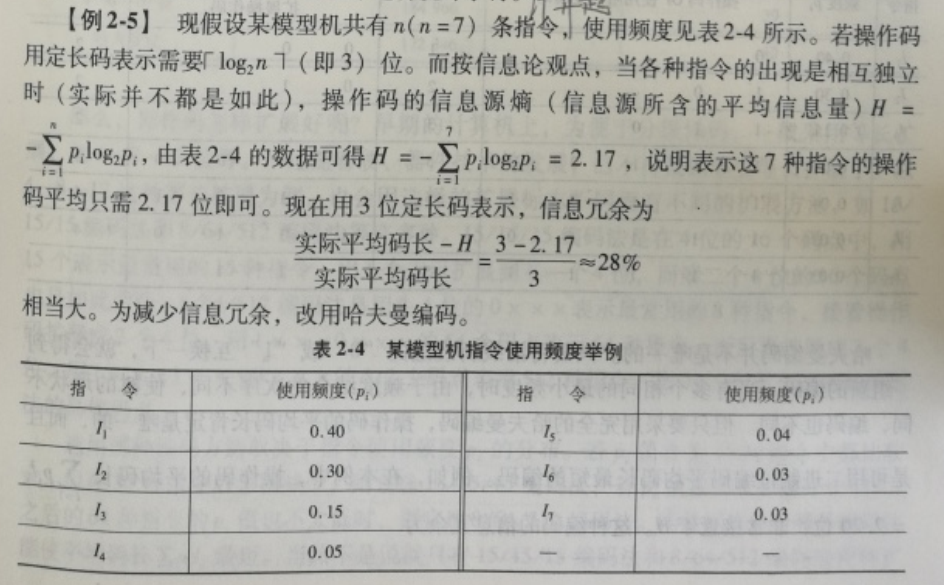结题思路：1、利用哈夫曼算法构造哈夫曼树。
构造哈夫曼树非常简单，将所有的节点放到一个队列中，用一个节点替换两个频率最低的节点，新节点的频率就是这两个节点的频率之和。这样，新节点就是两个被替换节点的父节点了。如此循环，直到队列中只剩下一个节点（树根）。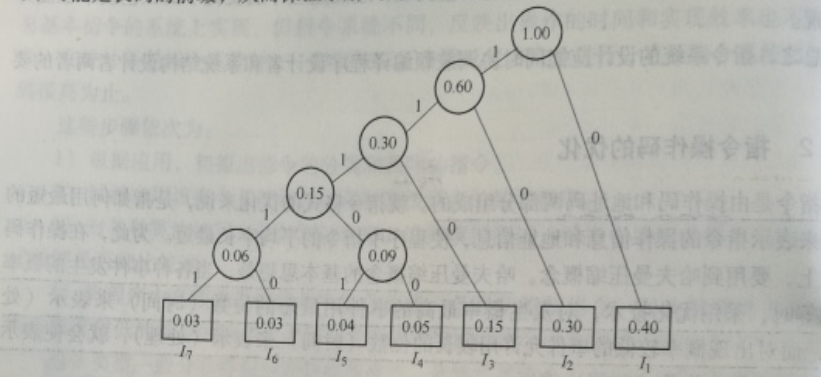2、根据绘制出的哈夫曼树，从根节点开始，沿线到达各频度指令所经过的代码序列就构成该频度指令的哈夫曼码，如下表所示：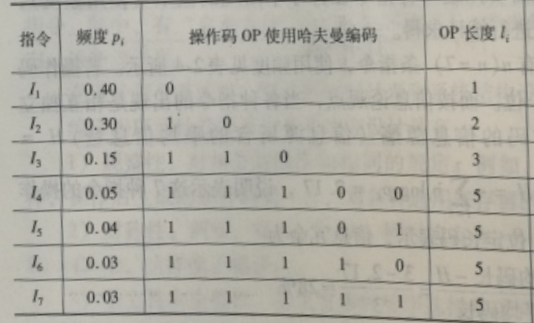3、根据编码表计算平均码长。
如下图，计算出来的平均码长为2.2位，非常接近于题目中给出的H，且这种编码的信息冗余仅有1.36%，远远小于三位定长码的信息冗余（28%）。所以，完全的哈夫曼编码是最优化的编码。
但是这种编码的码长种类太多。如上表，7种指令就出现了4种码长，长度有1有2有3有5，不规整，不易于编码。为此，结合用一般的二进制编码，在哈夫曼编码的基础上进行扩展。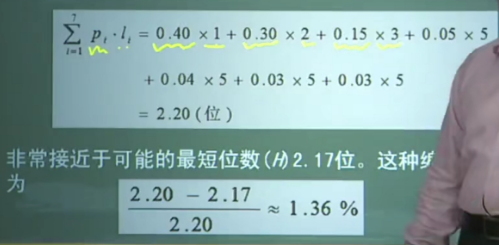## 扩展操作码编码

扩展操作码编码是介于定长二进制编码和完全的哈夫曼编码之间的一种编码方式，操作码不是定长的，但只有有限的几种码长。如上述题目，如果要求只能用固定两种码长，表示7条指令，应该使用什么编码方案？

最优的情况，肯定是尽可能用短的。所以我们先从最短码长开始考虑。

码长为1的情况，此时可以只能表示2条指令(2的1次方:0和1)，拿出1条用来扩展，扩展出几位呢？
1位，又可以表示2条，（2-1）+2=3<7，不足表示；
2位，又可以表示4条，（2-1）+4=5<7，不足表示；
3位，1+8=9>7，满足条件。
第一种方案出来了，码长为1的有1条，码长为4的有6条，平均码长=10.4+4（…）=2.8
注意：不足表示的情况，其实还可以继续扩展，但是这样就不符合两种码长的限制了。

码长为2的情况，此时可以只能表示4条指令（2的2次方:00，01，10，11），3条占用，拿出1条用来扩展，扩展出几位呢？
1位，又可以表示2条，（4-1）+2=5<7，不足表示；
2位，又可以表示4条，（4-1）+4=7=7，满足条件。
第二种方案出来了，码长为2的有3条，码长为4的有4条，平均码长=2*（0.4+0.3+0.15）+4*（…）=2.3

码长为3的情况，此时可以表示8条指令（2的3次方:000，001，010，011，100，101，110，111），足以表示，但是就不符合两种码长的要求，所以直接pass。而且之后的码长>3的情况，也都不符合。

综上，目前，我们只有两种方案，只需要比较哪个平均码长最短既可以了。
结论，最优编码方案：按照指令使用频度值将指令分为两组，频度较高的3条指令I1、I2、I3采用2位操作码编码表示，留下一个2位编码作为扩展标志，扩展出2位，用来编码其余频度较低的4条指令。平均码长为2.3。（很显然，扩展操作码编码的平均码长是大于哈夫曼编码的，冗余更多，浪费了一些，但是规整。硬件实现比较方便）

具体编码方案如下：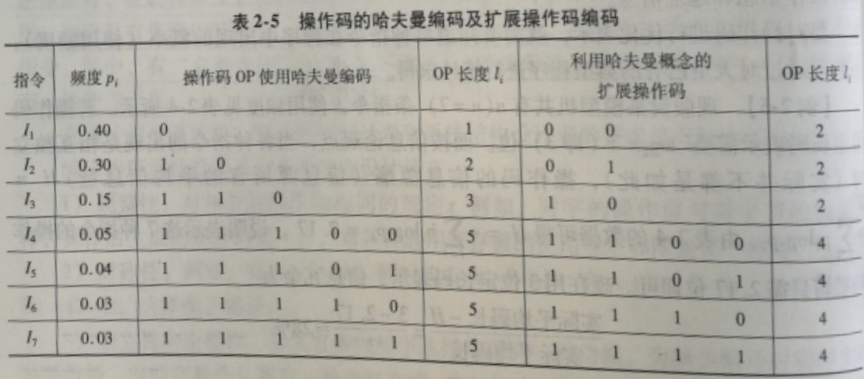热爱编程的小水怪，欢迎关注。有错请指出，一起加油。

展开全文•   使用编程工具编写一个程序，对一组指令进行霍夫曼编码，并输出最后的编码结果以及对指令码的长度进行评价，与扩展操作码和等长编码进行比较。 例如： 有一组指令的操作码共分七类，它们出现概率如下表所示。 ...

# 一、实验目的

了解和掌握指令编码的基本要求和基本原理

# 二、实验内容

使用编程工具编写一个程序，对一组指令进行霍夫曼编码，并输出最后的编码结果以及对指令码的长度进行评价，与扩展操作码和等长编码进行比较。

例如： 有一组指令的操作码共分七类，它们出现概率如下表所示。

指令P1P2P3P4P5P6P7
出现概率0.450.300.150.050.030.010.01

对此组指令进行 huffman 编码如下图所示：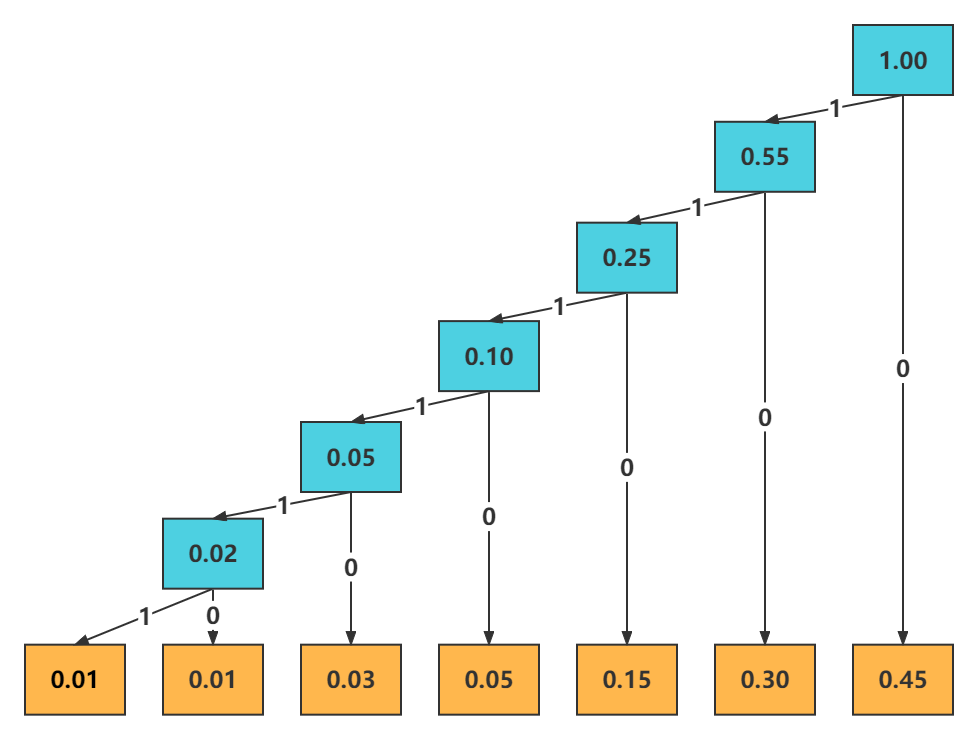最后得到的 huffman 编码如下表所示：

指令P1P2P3P4P5P6P7
出现概率0.450.300.150.050.030.010.01
huffman编码010110111011110111110111111
编码长度1234566

huffman 编码长度为： 0.45 x 1 + 0.30 x 2 + 0.15 x 3 + 0.05 x 4 + 0.03 x 5 + 0.01 x 6 + 0.01 x 6 = 1.97

要对指令的操作码进行 huffman 编码，只要根据指令的各类操作码的出现概率构造 huffman 树再进行 huffman 编码。此过程的难点在于构造 huffman 树，进行 huffman 编码只要对所生成的 huffman 树进行中序遍历即可完成编码工作。

# 三、实验步骤

观察上图，不难看出构造 huffman 树所要做的工作：

1. 先对各指令操作码的出现概率进行排序，构造一个有序链表。
2. 再取出两个最小的概率节点相加，生成一个生的节点加入到链表中，同时从两表中删除此两个节点。
3. 在对链表进行排序，链表是否只有一个节点，是则 huffman 树构造完毕，否则继续做步骤 2 的操作。
4. 为此设计一个工作链表、huffman 树节点、huffman 编码表节点。

# 四、实验结果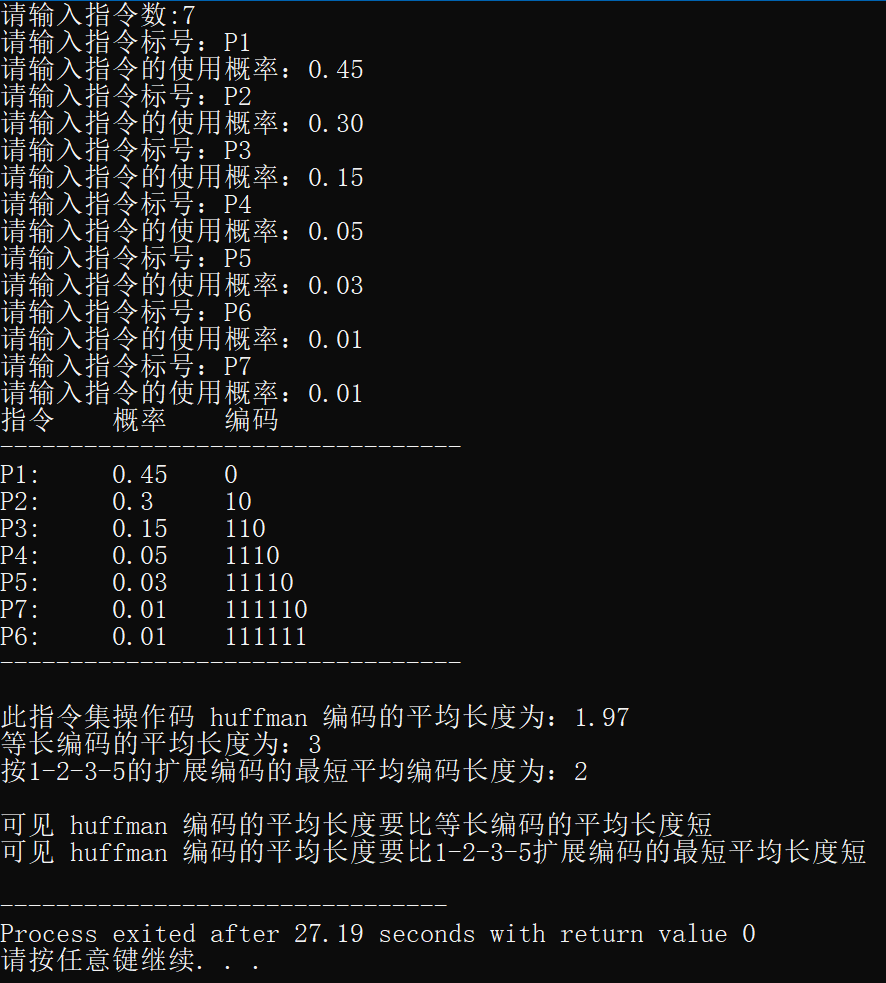# 五、实验代码

#include<iostream>
#include<cmath>
#include<cstring>

using namespace std;

const int N = 8; // huffman编码最大长度
class huff_p {
public:
huff_p* r_child;  // 大概率的节点
huff_p* l_child;  // 小概率的节点
char op_mask;  // 指令标号
float p;          // 指令使用概率
};

class f_min_p{
public:
f_min_p* next;
char op_mask;   // 指令标号
float p;           // 指令使用概率
huff_p* huf_p;
};

// huff_man code
class huff_code{
public:
huff_code* next;
float p;
char code[N];      // huffman 编码
};

f_min_p* input_instruct_set(); // 输入指令集子模块；
huff_p* creat_huffman_tree(f_min_p* head); // 构造huffman树;
f_min_p* fin_min(f_min_p* h);
f_min_p* del_min(f_min_p* h,f_min_p* p);
void insert_n(f_min_p* h,f_min_p* p);
huff_p*  creat_huffp(f_min_p* p);
void creat_huffman_code(huff_p* h1,huff_code* h);// 生成 huffman 编码;
void r_find(huff_p* p1,char code[],int i,huff_code* h);
void output_huffman(huff_code* head);// 输出huffman编码;
void cal_sort_length(huff_code* head);// 计算指令用huffman编码的平均编码字长
void print(huff_p* h1);
int main() {
f_min_p *h, *h1;
huff_p *root;

h = input_instruct_set();
h1 = h;
root = creat_huffman_tree(h1);

head = new huff_code;

while (pl) {
pl = pl->next;
}
}

f_min_p* input_instruct_set() {
f_min_p* h;
h = new f_min_p;
h->next = NULL;
h->huf_p = NULL;
int n;
cout << "请输入指令数:";
cin >> n;
cout << "请输入指令标号：";
cout << "请输入指令的使用概率：";
cin >> h->p;

f_min_p* point;
f_min_p* p1 = head;

for (int i = 0; i < n-1; i++) {
point = new f_min_p;
cout << "请输入指令标号：";
cout << "请输入指令的使用概率：";
cin >> point->p;
point->huf_p = NULL;
point->next = p1->next;
p1->next = point;
p1 = point;
}
}

huff_p* creat_huffman_tree(f_min_p* h) {
f_min_p *h1, *min1, *min2, *comb;
huff_p* head, *rd, *ld, *parent;
h1 = h;

min1 = fin_min(h1);
ld = creat_huffp(min1);
h1 = del_min(h1, min1);

if (h1->next) {
min2 = fin_min(h1);
} else {
min2 = h1;
}

rd = creat_huffp(min2);
comb = new f_min_p;
comb->next = NULL;
comb->p = rd->p + ld->p;

parent = creat_huffp(comb);

insert_n(h1, comb);
if (h1->next != NULL) {
h1 = del_min(h1, min2);
}

parent->l_child = ld;
parent->r_child = rd;

comb->huf_p = parent;

while (h1->next != NULL) {
min1 = fin_min(h1);
if (min1->huf_p == NULL) {
ld = creat_huffp(min1);
} else {
ld = min1->huf_p;
}
h1 = del_min(h1, min1);

if (h1->next) {
min2=fin_min(h1);
} else {
min2=h1;
}
if (min2->huf_p == NULL) {
rd = creat_huffp(min2);
} else {
rd = min2->huf_p;
}
comb = new f_min_p;
comb->next = NULL;
comb->p = rd->p + ld->p;
parent = creat_huffp(comb);
if (h1 != NULL) {
insert_n(h1, comb);
}

if (h1->next != NULL) {
h1 = del_min(h1, min2);
}
if (h1->next == NULL && ld->p < rd->p) {
huff_p* tmp = ld;
ld = rd;
rd = tmp;
}
parent->l_child = ld;
parent->r_child = rd;
comb->huf_p = parent;

if (h1->next == NULL) {
break;
}
}
delete comb;
}

f_min_p* fin_min(f_min_p* h) {
f_min_p *h1, *p1;
h1 = h;
p1 = h1;
float min = h1->p;
h1 = h1->next;
while (h1) {
if (min > (h1->p)) {
min = h1->p;
p1 = h1;
}
h1 = h1->next;
}
return p1;
}

f_min_p* del_min(f_min_p *h,f_min_p *p) {
f_min_p *p1, *p2;
p1 = h;
p2 = h;
if (h == p) {
h = h->next;
delete p;
} else {
while (p1->next != NULL) {
p1 = p1->next;
if (p1 == p) {
p2->next = p1->next;
delete p;
break;
}
p2 = p1;
}
}
return h;
}

void insert_n(f_min_p *h, f_min_p *p1) {
p1->next = h->next;
h->next = p1;
}

huff_p* creat_huffp(f_min_p* d) {
huff_p* p1;
p1 = new huff_p;
p1->l_child = NULL;
p1->r_child = NULL;
p1->p = d->p;
return p1;
}

void r_find(huff_p* p1, char code[], int i, huff_code* h) {
if (p1->l_child) {
code[i] = '1';
r_find(p1->l_child, code, i+1, h);
}
if (p1->op_mask != '\0') {
huff_code* p2 = new huff_code;
p2->p = p1->p;
int j = 0;
for (; j < i; j++) {
p2->code[j] = code[j];
}
p2->code[j] = '\0';
p2->next = h->next;
h->next = p2;
}
if (p1->r_child) {
code[i] = '0';
r_find(p1->r_child, code, i+1, h);
}
delete p1;
}

void creat_huffman_code(huff_p* h1, huff_code* h) {
int i = 0;
char code[N] = {'\0'};
r_find(h1, code, i, h);
}
void output_huffman(huff_code* head) {
huff_code* h = head->next;
cout << "指令\t" << "概率\t" << "编码" << endl;
cout<<"---------------------------------"<<endl;
while (h) {
cout << h->op_mask << ":\t" << h->p << "\t" << h->code << endl;
h = h->next;
}
cout << "---------------------------------" << endl;
cout << endl;
}
void cal_sort_length(huff_code* head) {
huff_code *h = head->next;
double j = 0;
float one_length = 0;
float per_length = 0;
float ext_length = 0;//按1-2-3-5扩展编码的最小长度为。

while (h) {
float length = 0;
int i = 0;
while (h->code[i] != '\0') {
length++;
i++;
}
one_length = h->p*length;
per_length = per_length + one_length;
h = h->next;
j++;
}
int i1 = int(j);
huff_code *p2 = head->next;
float* p_a = new float[i1];
//sort指令概率
int i0 = 0;
while (p2) {
p_a[i0++] = p2->p;
p2 = p2->next;
}
float max, temp;
int l;
for (int s = 0; s < i1; s++) {
max = p_a[s];
l = s;
for (int k = s+1; k < i1; k++) {
if (max<p_a[k]) {
max = p_a[k];
l = k;
}
}
temp = p_a[s];
p_a[s] = max;
p_a[l] = temp;
}
//计算1-2-3-5扩展编码的最短平均长度
float* code_len = new float[i1];
code_len = 1;
code_len = 2;
code_len = 3;
code_len = 5;
for (int i = 4; i < j; i++) {
code_len[i]=5;
}
l = 0;
while (l<i1) {
ext_length = ext_length + code_len[l]*p_a[l];
l++;
}

//计算等长编码平均长度；
int q_length = ceil(log10(j)/log10(2));

cout << "此指令集操作码 huffman 编码的平均长度为：" << per_length<<endl;
cout << "等长编码的平均长度为：" << q_length << endl;
cout << "按1-2-3-5的扩展编码的最短平均编码长度为：" << ext_length;
cout << endl;
cout << endl;
if (q_length > per_length) {
cout << "可见 huffman 编码的平均长度要比等长编码的平均长度短" << endl;
} else {
cout << "huffman 编码有问题请仔细查看算法，以及输入的指令集的概率之和是否大于1。" << endl;
}
if (ext_length>per_length) {
cout << "可见 huffman 编码的平均长度要比1-2-3-5扩展编码的最短平均长度短" << endl;
} else {
cout << "huffman 编码有问题请仔细查看算法，以及输入的指令集的概率之和是否大于1。" << endl;
}
}

展开全文huffman编码
• 根据哈夫曼树读出对应数据的哈夫曼编码 读出的过程我选择的是栈，从叶子节点迭代到根节点，出栈的时候字符就是正确的顺序了 具体代码 节点对象 public class HuffmanTreeNode { // 双亲节点 ...

# 步骤

1. 根据给出的数据和权重，构造完整的哈夫曼树
2. 根据哈夫曼树读出对应数据的哈夫曼编码
1. 读出的过程我选择的是栈，从叶子节点迭代到根节点，出栈的时候字符就是正确的顺序了

# 具体代码

节点对象

public class HuffmanTreeNode {

// 双亲节点
HuffmanTreeNode parentNode;

// 左孩子节点
HuffmanTreeNode leftChildNode;

// 右孩子结点
HuffmanTreeNode rightChildNode;

// 双亲节点索引
Integer parentIndex = 0;

// 左孩子节点索引
Integer leftChildIndex = 0;

// 右孩子节点索引
Integer rightChildIndex = 0;

// 值
Object value;

// 权重
Object weight;

public HuffmanTreeNode getParentNode() {
return parentNode;
}

public void setParentNode(HuffmanTreeNode parentNode) {
this.parentNode = parentNode;
}

public HuffmanTreeNode getLeftChildNode() {
return leftChildNode;
}

public void setLeftChildNode(HuffmanTreeNode leftChildNode) {
this.leftChildNode = leftChildNode;
}

public HuffmanTreeNode getRightChildNode() {
return rightChildNode;
}

public void setRightChildNode(HuffmanTreeNode rightChildNode) {
this.rightChildNode = rightChildNode;
}

public Object getValue() {
return value;
}

public void setValue(Object value) {
this.value = value;
}

public Object getWeight() {
return weight;
}

public void setWeight(Object weight) {
this.weight = weight;
}

public Integer getParentIndex() {
return parentIndex;
}

public void setParentIndex(Integer parentIndex) {
this.parentIndex = parentIndex;
}

public Integer getLeftChildIndex() {
return leftChildIndex;
}

public void setLeftChildIndex(Integer leftChildIndex) {
this.leftChildIndex = leftChildIndex;
}

public Integer getRightChildIndex() {
return rightChildIndex;
}

public void setRightChildIndex(Integer rightChildIndex) {
this.rightChildIndex = rightChildIndex;
}

public HuffmanTreeNode(HuffmanTreeNode parentNode, HuffmanTreeNode leftChildNode, HuffmanTreeNode rightChildNode, Integer parentIndex, Integer leftChildIndex, Integer rightChildIndex, Object value, Object weight) {
this.parentNode = parentNode;
this.leftChildNode = leftChildNode;
this.rightChildNode = rightChildNode;
this.parentIndex = parentIndex;
this.leftChildIndex = leftChildIndex;
this.rightChildIndex = rightChildIndex;
this.value = value;
this.weight = weight;
}

public HuffmanTreeNode(Object value, Object weight) {
this.value = value;
this.weight = weight;
}

public HuffmanTreeNode(Integer parentIndex, Integer leftChildIndex, Integer rightChildIndex, Object value, Object weight) {
this.parentIndex = parentIndex;
this.leftChildIndex = leftChildIndex;
this.rightChildIndex = rightChildIndex;
this.value = value;
this.weight = weight;
}

public HuffmanTreeNode(HuffmanTreeNode parentNode, HuffmanTreeNode leftChildNode, HuffmanTreeNode rightChildNode, Object value, Object weight) {
this.parentNode = parentNode;
this.leftChildNode = leftChildNode;
this.rightChildNode = rightChildNode;
this.value = value;
this.weight = weight;
}

public HuffmanTreeNode() {
}

@Override
public String toString() {
return "HuffmanTreeNode{" +
"parentNode=" + parentNode +
", leftChildNode=" + leftChildNode +
", rightChildNode=" + rightChildNode +
", parentIndex=" + parentIndex +
", leftChildIndex=" + leftChildIndex +
", rightChildIndex=" + rightChildIndex +
", value=" + value +
", weight=" + weight +
'}';
}
}

具体操作代码

public class HuffmanTreeDemo {

public static void main(String[] args) {

// 创建存储数组，大小为2n-1
List<HuffmanTreeNode> l =new ArrayList(7*2-1);

// 创建哈夫曼树
createHuffmanTree(l,7*2-1);

// 根据哈夫曼树读取哈夫曼编码
Stack<String> codeStack = new Stack();
Set<Map<String,String>> result = new HashSet<>();

for (int i=0;i<l.size();i++) {
HuffmanTreeNode huffmanTreeNode = l.get(i);

// 叶子节点才是我们需要的
if(huffmanTreeNode.getLeftChildIndex() == 0 && huffmanTreeNode.getRightChildIndex() == 0){

// 循环插入
stackPush(l, codeStack, i, huffmanTreeNode);

// 将栈中的字符串组合成一个完整的编码
StringBuilder stringBuilder = new StringBuilder();
while (!codeStack.isEmpty()){
stringBuilder.append(codeStack.pop());
}

// 存储编码
Map<String,String> map = new HashMap<>();
map.put(huffmanTreeNode.getValue().toString(), stringBuilder.toString());
}
}

// 打印哈弗曼树
for (int i=0;i< l.size();i++) {
System.out.println("index:"+i+"|||||||||||"+l.get(i));
}

// 打印哈夫曼编码
Iterator<Map<String, String>> iterator = result.iterator();
while(iterator.hasNext()){
System.out.println(iterator.next());
}
}

private static void stackPush(List<HuffmanTreeNode> l, Stack<String> codeStack, int i, HuffmanTreeNode huffmanTreeNode) {
// 双亲节点
HuffmanTreeNode parentNode = l.get(huffmanTreeNode.getParentIndex());

// 判断当前节点是双亲的哪个孩子结点，左0右1
if(parentNode.getRightChildIndex() == i) {
codeStack.push("1");
}else {
codeStack.push("0");
}

// 如果双亲节点不为0，说明还有双亲节点，需要递归查找
if(parentNode.getParentIndex() != 0){
stackPush(l,codeStack,huffmanTreeNode.getParentIndex(),parentNode);
}
}

private static void createHuffmanTree(List<HuffmanTreeNode> l,Integer maxIndex) {
while (true) {
// 最小权重的索引
Integer minIndex = 0;
// 第二小权重索引
Integer minIndex1 = 0;

// 最小权重值
BigDecimal minValue = BigDecimal.ZERO;
// 第二小权重值
BigDecimal minValue1 = BigDecimal.ZERO;

// 循环查找两个最小的权重的结点
for (int i = 0; i < l.size(); i++) {
// 双亲节点不为0的说明已经参加过合并，不再进行操作
if (l.get(i).getParentIndex() == 0) {
// 两个最小的值判断原理，永远将两个中较大的一个拿出作比较
if (minValue == BigDecimal.ZERO || minValue.compareTo((BigDecimal) l.get(i).getWeight()) > 0) {
// 存储小值
minValue = (BigDecimal) l.get(i).getWeight();
minIndex1 = minIndex;
minIndex = i;

// 如果另一个为0或者比第一个大，那么就交换两个的值
if(minValue1 == BigDecimal.ZERO || minValue1.compareTo(minValue) >= 0) {
BigDecimal temporary = minValue;
minValue = minValue1;
minValue1 = temporary;
}
}
}
}

// 创建新的结点
l.get(minIndex).setParentIndex(l.size() - 1);
l.get(minIndex1).setParentIndex(l.size() - 1);

// 如果数组的长度和我们规定的最大值一样，说明结束了
if (l.size() == maxIndex) {
break;
}
}
}
}

输出结果

index:0|||||||||||HuffmanTreeNode{parentNode=null, leftChildNode=null, rightChildNode=null, parentIndex=12, leftChildIndex=0, rightChildIndex=0, value=A, weight=0.4}
index:1|||||||||||HuffmanTreeNode{parentNode=null, leftChildNode=null, rightChildNode=null, parentIndex=11, leftChildIndex=0, rightChildIndex=0, value=B, weight=0.3}
index:2|||||||||||HuffmanTreeNode{parentNode=null, leftChildNode=null, rightChildNode=null, parentIndex=10, leftChildIndex=0, rightChildIndex=0, value=C, weight=0.15}
index:3|||||||||||HuffmanTreeNode{parentNode=null, leftChildNode=null, rightChildNode=null, parentIndex=8, leftChildIndex=0, rightChildIndex=0, value=D, weight=0.05}
index:4|||||||||||HuffmanTreeNode{parentNode=null, leftChildNode=null, rightChildNode=null, parentIndex=8, leftChildIndex=0, rightChildIndex=0, value=E, weight=0.04}
index:5|||||||||||HuffmanTreeNode{parentNode=null, leftChildNode=null, rightChildNode=null, parentIndex=7, leftChildIndex=0, rightChildIndex=0, value=F, weight=0.03}
index:6|||||||||||HuffmanTreeNode{parentNode=null, leftChildNode=null, rightChildNode=null, parentIndex=7, leftChildIndex=0, rightChildIndex=0, value=G, weight=0.03}
index:7|||||||||||HuffmanTreeNode{parentNode=null, leftChildNode=null, rightChildNode=null, parentIndex=9, leftChildIndex=6, rightChildIndex=5, value=, weight=0.06}
index:8|||||||||||HuffmanTreeNode{parentNode=null, leftChildNode=null, rightChildNode=null, parentIndex=9, leftChildIndex=4, rightChildIndex=3, value=, weight=0.09}
index:9|||||||||||HuffmanTreeNode{parentNode=null, leftChildNode=null, rightChildNode=null, parentIndex=10, leftChildIndex=8, rightChildIndex=7, value=, weight=0.15}
index:10|||||||||||HuffmanTreeNode{parentNode=null, leftChildNode=null, rightChildNode=null, parentIndex=11, leftChildIndex=9, rightChildIndex=2, value=, weight=0.30}
index:11|||||||||||HuffmanTreeNode{parentNode=null, leftChildNode=null, rightChildNode=null, parentIndex=12, leftChildIndex=10, rightChildIndex=1, value=, weight=0.60}
index:12|||||||||||HuffmanTreeNode{parentNode=null, leftChildNode=null, rightChildNode=null, parentIndex=0, leftChildIndex=11, rightChildIndex=0, value=, weight=1.00}
{A=1}
{C=001}
{G=00010}
{B=01}
{F=00011}
{D=00001}
{E=00000}

结果输出的哈夫曼树样子和王卓老师视频中的样子不太一样，属于是同分异构体的关系。

左0右1即可得出上述结果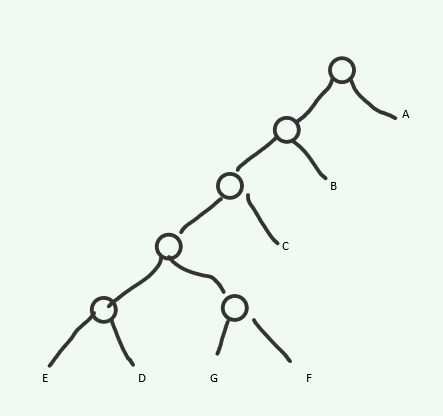展开全文数据结构 霍夫曼树
• 一、什么是哈夫曼编码？ 1.文件编码 文件是由字符构成的，ACSII中大概包含100个可打印的字符，每一个字符都有其特定的编码，扩展ACSII为8位，也就是说不同的字符都需要用8位二进制位来编码，这是一种等长。 ...
• 文章目录对于可变长指令指令设计要点例题巩固 ...有点儿像哈夫曼编码 指令设计要点 1)不允许短码是长码的前缀，即短操作码不能与长操作码的前面部分的代码相同。 2）各指令的操作码一定不能重复。 例题巩固 ...
• ## 哈夫曼编码习题

万次阅读 多人点赞 2019-11-07 19:57:45
假设用于通信的电文仅由8个字母组成，字母在电文中出现的...请为这8个字母设计哈夫曼编码。 表格形式： NO. data parent Lchild Rchild 0 0.07(A) 10 NULL NULL 1 0.19(B) 12 ...三叉链表 数据结构
• 哈夫曼编码是一种不等长编码，常用字符编码短，不常用字符编码长。 不等长编码需要解决两个关键问题： （1）编码尽可能短（最长的编码最短） （2）不能有二义性（前缀特性：一个编码不能是另一个编码的前缀） ...霍夫曼树 数据结构
• ## 4-2扩展操作码

千次阅读 2020-12-01 19:40:35
学习目标： 做题 学习内容： 概念： 1、 操作码、地址码的概念 2、 根据地址码数目不同分类 3、 根据指令长度分类 4、 根据操作码的长度不同分类 ...二地址指令为15条，将1111 1111留作扩展操作码
• 文章目录扩展操作码扩展操作码举例设计扩展操作码需注意：设计扩展操作码例题：指令操作码操作码分类：定长操作码：扩展操作码(不定长操作码) ： 扩展操作码 指令由操作码和若干个地址码组成。 PS：先回顾一下指令字...java python 数据结构 大数据
• ## 哈夫曼树及编码讲解及例题

万次阅读 多人点赞 2019-04-19 16:17:11
哈弗曼树及编码 哈弗曼树算法 第一步： 初始化n个单节点的树，并为它们表上字母中的字符。把每个字符的概率记在树的根中，用来指出树的权重(更一般地说，树的权重等于树中所有叶子的概率之和)。 第二部： 重复下面的...哈弗曼编码 贪心
• 　哈夫曼（Huffman）编码算法是基于二叉树构建编码压缩结构的，它是数据压缩中经典的一种算法。算法根据文本字符出现的频率，重新对字符进行编码。因为为了缩短编码的长度，我们自然希望频率越高的词，编码越短，...
• 哈夫曼编码是可变字长编码(VLC)的一种。该方法完全依据字符出现概率来构造异字头的平均长度最短的码字，有时称之为最佳编码。 简言之就是，我们的一段话中，每个字母都会有它的出现频率，如果出现频率很高的字母，...
• 计算机系统结构-第二章自考练 习题答案 第二... 哈夫曼编码 2浮点数尾数基值 r m=16 除尾符之外的尾数 机器位数为 8 位时可表示的规格化最大尾 数值为 D A. 1/2 B. 15/16 C. 1/256 D. 255/256 3. 自定义数据表示包括 标
• 1 引言 对前缀编码进行解码时，最重要的问题是如何快速的确定...范式哈夫曼编码具有数字序列属性，因而能通过如下算法确定码字的长度： int len = 1; int code = bs.ReadBit(); while(code >= first[len])...压缩 范式Huffman
• 3 指令系统 此处每年必考，以选择填空的形式出现，主要掌握扩展指令码的分配(实质上就是操作码去占用地址码实现扩展的过程)，寻址方式主要是间接寻址、变址寻址和相对寻址的计算问题，尤其是相对寻址在执行跳转...
• 知识点及要求1)能够正确读写文件2)统计文本文件中各字符频率3)编码：构造哈夫曼树，得到各字符的哈夫曼编码4)按照哈夫曼编码将文件A翻译为Huffman编码文件B。5)译码：对文件B进行译码，得到文件C6)比对文...
• ## 求霍夫曼扩展编码

万次阅读 2013-09-11 16:37:51
某计算机有10条指令，它们的使用频率分别为 0.30,0.20, 0.16, 0.09, 0.08...(2)用扩展编码法对操作码进行编码，限两种操作码长度，并使平均码长最短。 (1) 霍夫曼编码的结果以及各编码的长度如下所示： 0.30 0.20
• 树的应用 等价类问题 哈夫曼树和哈夫曼编码 图 图的概念 图的存储及基本操作 邻接矩阵法 邻接表法 图的遍历 深度优先搜索 广度优先搜索 图的基本应用及其复杂度分析 最小(代价)生成树 最短路径 拓扑排序 关键路径 ...计算机算法基础
• huffman编码C语言实验报告今日推荐 180份文档 2014...4页 1下载券 安卓版100 doors 2攻略1... 3页 1下载券 《逃脱本色》doors...。语文教育实习日志,40篇 21页 1下载券 教师实习日志 11页 1下载券 销售实习日记40篇...
• 具体地，围绕哈夫曼编码展开，进行编码需要： 读取文件并统计“数据元”的次数(代表频率) 这里数据元不能简单只作为字符处理，因为想的是能压缩任意格式，所以 我们要采用二进制方式处理信息，将二数据结构
• 1 哈夫曼编码综述在计算机科学和信息论，哈夫曼编码是一种特殊类型的最优前缀(prefix code)，通常用于无损数据压缩(英文文本，更一般地说 ASCII 位于 0-255 位的文本)。哈夫曼编码是一种变长编码，相比使用定长...
• 简单快速的哈夫曼编码 介绍 本文描述在网上能够找到的最简单，最快速的哈夫曼编码。本方法不使用任何扩展动态库，比如STL或者组件。只使用简单的C函数，比如：memset，memmove，qsort，malloc，realloc和memcpy...byte
• 这是我大一写过的一个小项目，现在大三，重新实现了一下。这是原来的链接可以看一下...扩展性、健壮性比原来更好，思路也更清晰了。当时只想花里胡哨，这次重心放在质量、功能上。 后续会不断改进它，直到它贴近实际。
• 一个简单的压缩软件，利用哈夫曼思想，构造哈夫曼编码，实现对文件的二进制压缩，以及解压，再利用MFC制作可视化操作界面，美化软件又简化文件操作。（各个步骤有解释可看） 软件主页面先看看 哈夫曼树结构 构造...数据结构
• 香农-范诺编码香农-范诺(Shannon-Fano)编码的目的是产生具有最小冗余的词(code word)。其基本思想是产生编码长度可变的词。词长度可变指的是，被编码的一些消息的符号可以用比较短的词来表示。估计词长度...
• 3.指令集操作分类 CISC 弊端: 指令集过于庞杂。 使用微程序技术降低了机器的处理速度。 指令系统过于庞大。 完善的中断控制导致动作繁多，设计复杂，研制周期长。 给芯片设计带来困难。 RISC 基本思想： 通过...
• ，因为编码结果是前缀，所以经过哈夫曼编码后的区间码字各不相同，都是唯一的，所以，用“区间码字+扩展值”就可以唯一标识一个distance码字。比如区间4和区间9的哈夫曼编码码字分别为二进制“0010”和“0111...deflate gzip 动态哈夫曼编码原理 构建哈夫曼树...